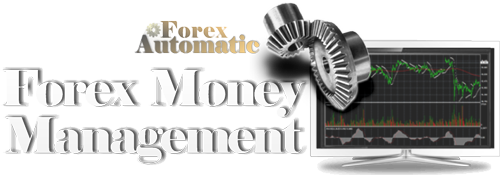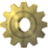# Automated Forex Money Management

Designing a simple and effective Money Management System to trade Forex or any other Financial Market.Effective and sophisticated Money Management counts at least 50% of the long-term success of any trader in any financial market. The lack of the right money management system may lead even experienced and very talented traders to great losses, in the long-run. Here are some key points/conclusions produced by this analysis.

The Key points:

◘ The Basic two Questions to be answered are i) when to Trade, and ii) How Much to Trade

◘ The Rate of Leverage must be always in balance with i) Profit/ Loss Ratio, ii) Trading Cost, and iii) Overall Risk Tolerance

◘ Portfolio Diversification is always crucial no matter if you are a Professional Investor or a Beginner Trader

◘ No Individual Trade should worth more than 1/1000 than the Trader’s Annual Income

◘ Trading Portfolio should worth less than the Trader’s Annual Income

The Two Basic Questions When Trading the Financial Markets

Long-run Profitable Trading requires the existence of a complete trading system. A complete trading system is a system that is able to answer two basic questions, at any given time:

1. When-to-Trade and When Not (Time)

2. How much to Trade each Time (Money)

So Money Management is all about time and money. The right time to trade is actually the subject of technical analysis and thus it will not be covered in this analysis. The second question “How much to Trade” is a clear Money Management issue and it will be analyzed below.

Incorporating Portfolio Diversification into any Money Management System

Anyone who is familiar with financial economics/investment knows that portfolio diversification is the golden rule of investing. “Don’t Put all your Eggs in the same Basket” can be translated as “Don’t put all your Money is the Same Trading Opportunity”. Portfolio Differentiation has proven to be the ideal method of investing in studies during periods of 100 years and more. Therefore we need to distribute our funds in many positions, not in a few positions. But in how many positions should we distribute our funds to? This question can be answered via a simple model:

Step-1) Calculate Total Annual Income

Step-2) Divide it by 1,000

Step-3) The Outcome is the Highest Amount of Money you Should Invest in a Single Trade

For example, if the Annual Income is 20,000 USD, the maximum individual trade should worth no more than 200 USD while for an Annual Income of 1 million USD, the maximum individual trade should worth no more than 10,000 USD.

Note that a trade worth just 200 USD may be leveraged many times as Forex traders are able to use high capital leverage to raise technically their funds. So actually the question how much to trade is almost the same as the question “How much leverage should be used in Each Trade?”.

Leverage is not a Trading Asset, it is a rather a Trading Liability

Online Forex Trading is characterized by two main facts i) enormous liquidity, and ii) by the ability to leverage the trading capital. The available leverage when trading Forex is ranging from 50:1 to even 500:1 or even more. That means that even a portfolio of 10,000 USD may trade millions of dollar in a single trade. It sounds like a great opportunity but actually, high leverage is also a great threat for any portfolio. That is because, the more trading leverage used, the more risk you must accept and the more trading cost you must pay.

“High Capital Leverage involves High Risk and High Transactional Cost”

The Leverage Formula

In a past article published on the partner TradingCenter.org, I have been referred to the leverage formula. The leverage formula is a simple formula to define the optimal level of leverage of any trade.

Leverage Formula = [ P/L Ratio x ( 1 / Spread ) x R ] %

Where:

■ (P/L) = Profit to Loss Ratio, or else Potential Profit Pips / Potential Loss Pips

■ Spread is the difference between Bid and Ask (Demand and Supply), it is measured in pips

■ (R) = Risk Tolerance Ratio is the ability of a trader to accept the risk

This simple formula combines:

1. Technical Analysis (To identify Profit / Loss pips)

2. Market conditions (Spread and Commissions in pips)

3. Money Management (Individual Ability to Accept Risk)

Therefore by solving this simple formula, we are able to answer the basic question of “How much Trading Leverage should we use each time we trade”.

Solving the Formula –Calculating all Components

i) Calculating Profit / Loss Ratio

The first component is the P/L ratio. Technical analysis can be used to provide information about what is the potential profit and loss each time we trade. In general for those who are trading using ‘Support & Resistance,’ the potential profit is the difference between the Current Price and the First Major Resistance, while the Potential Loss is the difference between the Current Price and the First Major Support Level.

Calculation:

■ Potential Profit = First Major Resistance – Current Price

■ Potential Loss = Current Price - First Major Support

For more advanced P/L calculations more support and resistance levels may be used, as follows:

-Averaging the 3 Closest Major Support and Resistance Levels:

■ Potential Profit = Average Price of the 3 Closest Major Resistance Levels – Current Price

■ Potential Loss = Current Price - Average Price of the 3 Closest Major Support Levels

ii) Spread and Commissions Charged in pips

This second component is trading cost. Trading cost includes:

-Commissions Charged

-Swap Charges (Curry Trading)

Calculation:

The calculation here is very simple as long as all charges are transformed into pips.

iii) Personal Ability to Accept Risk

This is a very important factor that usually is not taken seriously enough.

Calculation:

a) Calculating Maximum Portfolio Value

Step-1) Measure the Total Value of your Portfolio

Step-2) Calculate your Total Annual Income

■ A trading portfolio should never be larger than the corresponding annual income, so Annual Income defines the highest value of any Trading Portfolio in our Formula (max 100%)

b) Calculating Maximum Risk Tolerance Ratio

Step-3) Divide the Value of your Trading Portfolio to your Annual Income

■ Risk Tolerance = [1 - (Value of Portfolio / Annual Income)]%

That means that if the value of the portfolio is 10,000 USD and the annual income is 30,000 USD then the Value of Portfolio / Annual Income = 0.33

Thus the Risk Tolerance Ratio may be calculated as:

Risk Tolerance Ratio = [1 - (Value of Portfolio / Annual Income)]% = [1-0.33]% = 0.67%

So the Risk Tolerance of this Portfolio worth 10,000 USD will be 67%.

Leverage Formula -Putting all the Pieces Together

■ Leverage Formula= [ P/L Ratio x ( 1 / Spread ) x R ] %

George Protonotarios

Financial Analyst / Investment Consultant

ForexAutomatic.comREAD MORE ON FOREX AUTOMATIC

 • COMPARE □ Compare Trade Systems □ Compare Platforms □ Compare Forex Brokers • GUIDES ► Automated Trading ► Forex Rollover Rate (swap) ► Automated Trade Strategies ► Intermarket Analysis